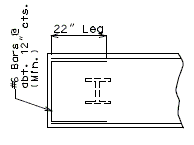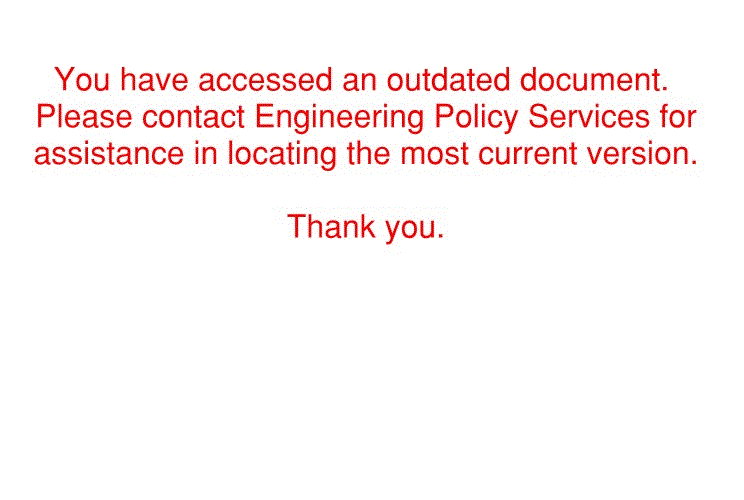# 751.32 Concrete Pile Cap Intermediate Bents

(diff) ← Older revision | Latest revision (diff) | Newer revision → (diff)

## 751.32.1 General

### 751.32.1.1 Material Properties

Concrete
Typically, shall consist of:
Class B Concrete (Substructure) $\,f'_{c}$= 3.0 ksi
$\,n$= 10

In addition, Class B-1 Concrete (Substructure) may also be used in special cases (See Project Manager). The following equations shall apply to both concrete classes:

Concrete modulus of elasticity:

$\,E_{c}=33000K_{l}w_{c}{\sqrt[{1.5}]{f'_{c}}}$Where:

 $\,w_{c}$= unit weight of non-reinforced concrete = 0.145 kcf $\,K_{l}$= correction factor for source of aggregate = 1.0

 Modulus of Rupture: For minimum reinforcement, $\,f_{r}=0.37{\sqrt {f'_{c}}}$For all other calculations, $\,f_{r}=0.24{\sqrt {f'_{c}}}$$\,{\sqrt {f'_{c}}}$is in units of ksi

Reinforcing Steel

 Minimum yield strength, $\,f_{y}$= 60.0 ksi Steel modulus of elasticity, $\,E_{s}$= 29000 ksi

## 751.32.2 Design

### 751.32.2.1 Limit States and Factors

In general, each component shall satisfy the following equation:

$\,Q=\sum \eta _{i}\gamma _{i}Q_{i}\leq \phi R_{n}=R_{r}$Where:

 $\,Q$= Total factored force effect $\,Q_{i}$= Force effect $\,\eta$= Load modifier $\,\gamma _{i}$= Load factor $\,\phi$= Resistance factor $\,R_{n}$= Nominal resistance $\,R_{r}$= Factored resistance

Limit States

The following limit states shall be considered for bent design:

STRENGTH – I
STRENGTH – III
STRENGTH – IV
STRENGTH – V
SERVICE – I
FATIGUE

See LRFD Table 3.4.1-1 and LRFD 3.4.2 for Loads and Load Factors applied at each given limit state.

Resistance factors

STRENGTH limit states, see LRFD 5.5.4.2
For all other limit states, $\,\phi$= 1.00

## 751.32.3 Details

### 751.32.3.1 General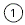Use 2'-6" minimum or as determined by the superstructure requirements or the minimum support length required for earthquake criteria (expansion joint bents only) (3" increments).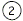= 2'-9" (Min.) for Wide Flange and Double-Tee Girders or,    3'-0" (Min.) for Prestressed Girders and Plate Girders.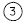Check the clearance of the anchor bolt well to the top of pile. Increase the beam depth if needed.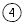If the depth at the end of the beam, due to the steps, exceeds 4~6", the beam bottom should be stepped or sloped.

### 751.32.3.2 Front Sheet

 Note: The following are details and dimensions for the Plan View on the Front Sheets. Details for unsymmetrical roadways will require dimensions tying Centerline Lane to Centerline Structure.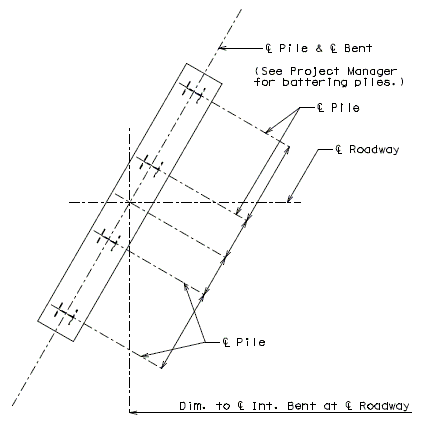## 751.32.4 Reinforcement

### 751.32.4.1 General

 Note: Locate #4 bars "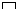" under bearings where required to maintain a 6" maximum spacing of combined stirrups. (#4 bars "" are not required for Double-Tee Structures.) When an expansion device is used at an intermediate bent, all reinforcement located entirely within the beam or extending into the beam shall be epoxy coated.

Reinforcement Under Bearings Transverse Beam Steps - Over 3"(also, Steps Accumulating Over 3")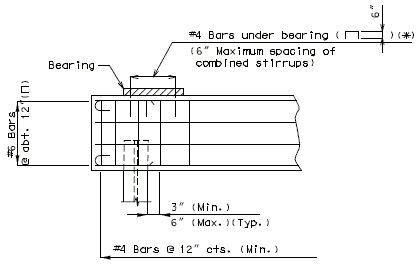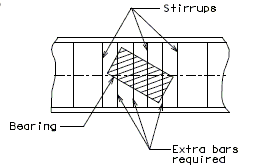* #4 Bars () not required for Double-Tee structures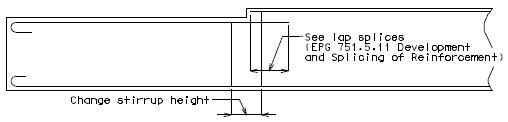Part Plan